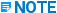# Copy Assembly - PDS - 2011 R1 - Reference

## FrameWorks Plus Programmer's Reference

PPMProduct
PDS
PPMCategory_custom
Reference

Syntax

copy_assem assem_num = I, delta = R, R, R
[ , n_copies = I ] [ , new_assem_nums = I, I, I, . . . n ]
[ , form_type = I ] ;

Usage

This command selects all of the elements written from within the PML program that are part of the specified assem_num (assembly number). The command then evaluates X, Y, and Z components for delta and copies the selected elements n_copies number of times; each time incrementing the element placement coordinates by delta. If new assembly numbers (new_assem_nums) are assigned, the new elements will reflect that. In other words, if three copies are to be made, each copy can have a different assembly number assigned to it.

You can have up to 50 copies of each assembly, but the new assembly numbers can only have three unique values. If you do not specify the assembly numbers for all of the copies, the last assembly number used will be used for the remainder of the copies. For example, if you say that n_copies = 6, new_assem_nums = 1, 2, 3, 1 then the first three copies will be numbered 1, 2, and 3, and the remaining three copies will use the number 1.

Limitations

The n_copies argument must be issued before new_assem_nums.Assemblies are not used in FrameWorks Plus. This command is available only for compatibility with ModelDraft PMLs.

Arguments

assem_num -- A valid assembly number (1 through 255) that has already been created in the PML program and has elements successfully placed in it.

delta -- The X, Y, Z increment should not all equal zero.

n_copies -- The number of copies must be greater than zero and less than or equal to 50.

new_assem_nums -- The list of new assembly numbers should be unique and greater than zero. There should be n_copies of new assembly numbers. There have to be as many integers as there are copies. For example, if you want 3 copies, you need 3 integers. (none)

form_type -- The report format type, which should be set to zero. This argument is not used by PML.

Example Syntax

• copy_assem assem_num = 1,
delta = 0,10,0;

• copy_assem assem_num = 10,
delta = 5,0,0,
n_copies = 5,
new_assem_nums = 11,12,13,14,15;

var_type = "int",
field_num = 24;

• start_assem assem_num = ladder_assem + 1;

• plc_column end1 = clearx, -cleary, 0,
end2 = clearx, -cleary, height;

• plc_column end1 = x + clearx, y - cleary, 0,
end2 = x + clearx, y - cleary, height;

• end_assem assem_num = ladder_assem + 1;

• start_assem assem_num = ladder_assem + 2;

• plc_beam end1 = clearx, -cleary, firststep,
end2 = x + clearx, y - cleary, firststep;

• copy_last_elem delta = 0,0,rungspace,
n_copies = rungcopies;

• end_assem assem_num = ladder_assem + 2;

• end_assem;